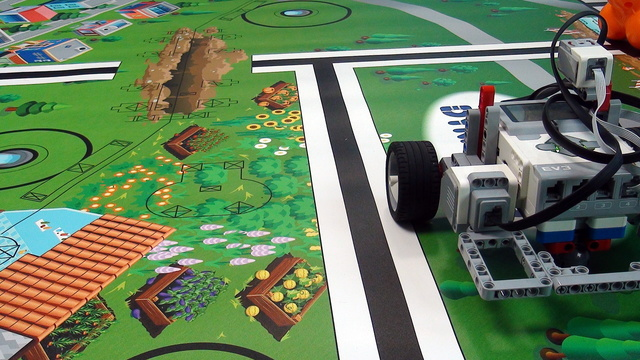# Keep the LEGO robot orientation straight. Proportional Part. Coefficients. ProPreview

We should multiply the error by a certain number and then add it to the steering of the LEGO Mindstorms Steering block. In this way, by changing the coefficient we change how much/fast should the proportional part influence the steering of the robot.

• #669
• 10 Jan 2018
• 3:43

### Influence of the coefficient

#### When the robot is oscillating too much - this means that the coefficient for the proportional part is too large. It should be reduced.

When the robot is not returning fast enough to the straight orientation - this means that the coefficient for the proportional part is too small. It should be increased.

### Program for keeping the robot straight with the Gyro Sensor (Proportional compensation)

Proportional implementation for keeping the LEGO Mindstorms robot straight. The program will take the value of the Mindstorms Gyro sensor and will apply this value to the steering block. This will make the robot steer in a direction that would put the robot in a straight position again.

### English

Previously we did this very small and interesting program for keeping the orientation of our robot straight and it was using the gyro sensor and it is a proportional algorithm where we just apply the value of the gyro sensor to the steering block. And if the value of the gyro sensor is like 10, this means that the steering will steer more to the left or more to the right and the larger the value, the more the steering. And there is one more thing that we have to mention and this is how to add a coefficient here to control how much should the gyro sensor apply to the steering. And this is the thing I would like to do in this video. What you should generally do it's a good practice is the following way: In this program you use the math block and it's a very simple equation we must do. And we take the value of the gyro sensor and we add it to the math block and then we multiply this value by something. And the result goes in the steering block. Now this is a very simple change but very powerful. The idea of the change is the following. If the gyro sensor detects an angle of 10 degrees for example then we apply these 10 degrees to the steering. If the gyro sensor detects 100 degrees, then we apply 100. So, we directly take the value of the gyro sensor and we directly apply to the steering. And this might be too much for the steering or it might be too little and this depends on the robot. So, what should happen for example if we detect with the gyro sensor a value of 2? And we would like to apply a steering of 10 to the steering block because this is the way our steering is constructed. What we should do is multiply this value by some coefficient and let's say this coefficient is 2. This means that if the gyro sensor detects 1, so this is the difference between moving forward and slightly to the right and if it detects a value of 1, we multiply this value by 2 and it will try to faster return to the straight position. It will much faster try to return to the straight position. If we have a value of let's say 10,

then even a very small change in the gyro sensor would make the robot turn very fast and try to return very fast to the straight position. And this is something that you should experiment with depending on your robot and the size of the field and the requirements of the competition that you are trying to achieve. So, it is important to have this coefficient. It could be just one or it could be even less that 1 let's say 0.3. And there is no golden rule here or no golden value. It is something that depends on the conditions. So, you should experiment and find the value that matches the best for your robot and the behavior that you would like to get from your robot.

## Courses and lessons with this Tutorial

This Tutorial is used in the following courses and lessons• 24
• 102:09
• 3• 5
• 0
• 0
• 3d_rotation 1